Open in App
Not now

## Related Articles

• CBSE Class 9 Syllabus (All Subjects)
• CBSE Class 9 Maths Notes
• CBSE Class 9 Science Revision Notes
• CBSE Class 9 Social Science Revision Notes

# Basic Constructions – Angle Bisector, Perpendicular Bisector, Angle of 60°

• Last Updated : 31 Aug, 2021

Most of the time we use diagrams while depicting the shapes and scenarios in mathematics. But they are not precise, they are just a representation of the actual shape without proper measurements. But when we are building something like a wooden table, or map of a building is to be constructed. It needs to be precise in measurements. For such cases, we need to learn to do some basic constructions. Let’s study them in detail.

### Introduction to Constructions

To draw polygons, angles, or circles some basic instruments are needed which are given in our geometry box.

A geometry box should contain the following instruments:

1. A ruler or graduated scale:

It should have centimetres and millimetres marked off on one side and the other side should have inches and their parts marked off on it.

2. Pair of Set Squares:

One should have 60°, 90° and 30° as its angles and the other one should have 90°, 45° and 45°.

3. Divider

Dividers have a jointed pair of legs, each leg has a sharp point. They can be used for scribing a circles and taking off and transferring the dimensions.

4. Compass

Compass is used for inscribing circles or arcs. As similar to dividers, they can also be used for taking off and transferring the dimensions.

5. Protractor

This instruments helps us in measuring the angles. It has degrees marked from 0 to 180 and from 180 to 0. It has readings from both left to right and right to left. Both the readings supplement each other.

For learning about the basic constructions we will mostly use three of them. Graduated Scale, Compass, and a Protractor. Let’s see some basic constructions.

### Basic Constructions

We know that a bisector is a line that divides anything into two equal parts, be it an angle or a line segment. Let’s see how to make bisectors of any given angle using our instruments from the geometry box.

### Construction 1: Angle Bisector

Let’s say we are given an angle PQR and the goal is to construct a bisector of the given angle.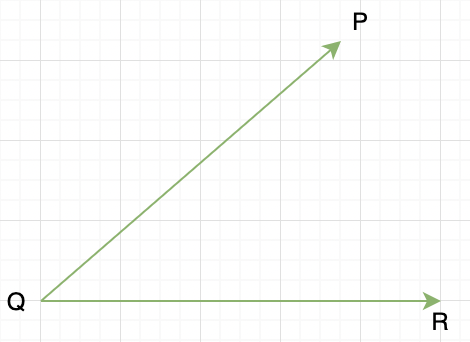Steps for Construction:

Step 1.  Take Q as centre and draw an arc that intersects the rays QP and QR with any radius. Let’s name the intersection points T and S.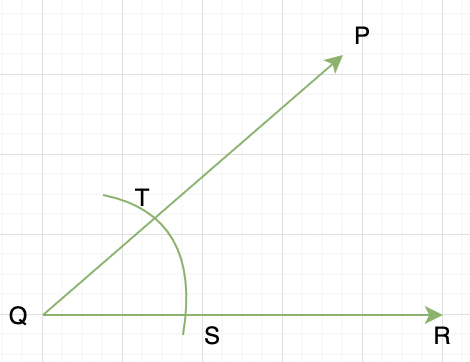Step 2. Now take T and S as the Centre and with the radius that is more than half of the length of TS. Draw arcs such that they intersect, let’s call that intersection U.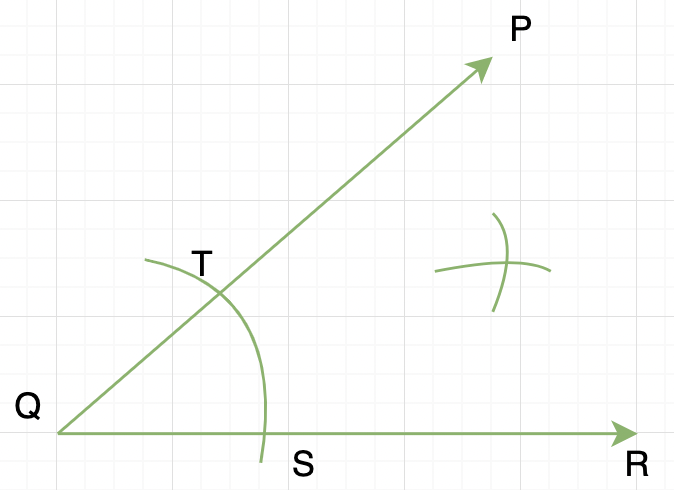Step 3. Now join QU, this is our required bisector.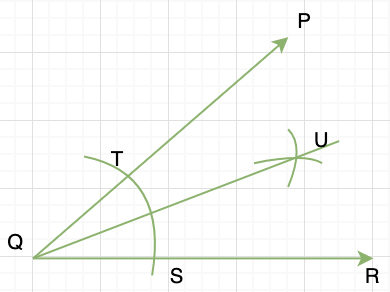### Construction 2: Perpendicular bisector of the given line.

A perpendicular bisector is a line that bisects the given line and is perpendicular to it. Given a line PQ, the goal is to construct a perpendicular bisector.

Steps for Construction:

Step 1. Let’s take P and Q as the centre and take any radius that more than half the length of PQ. Now draw arcs on both sides of the line PQ and let them intersect at A and B respectively.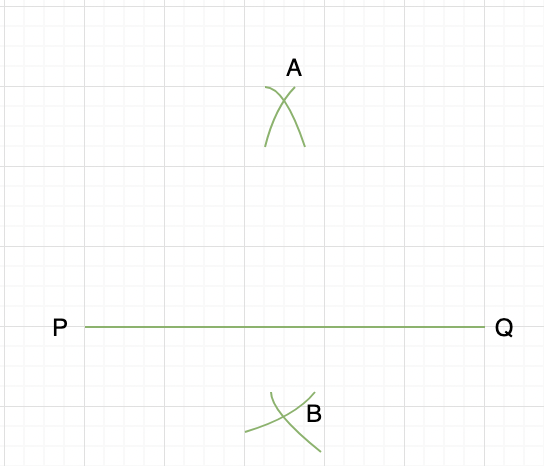Step 2. Now Join AB and let it intersect at M on PQ. This is our required perpendicular bisector.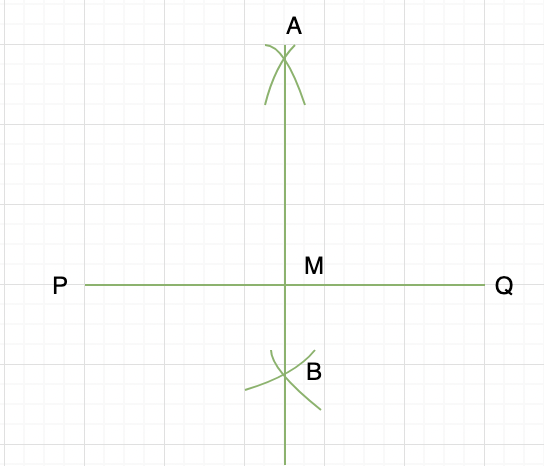### Construction 3: An angle of 60°

The goal in this construction is to construct an angle of 60° from a given ray PQ.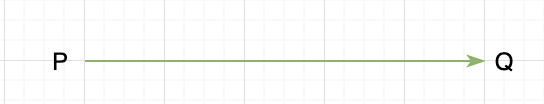Steps of Construction:

Step 1. With P as the centre and some arbitrary radius, construct a circular arc that intersects PQ at S.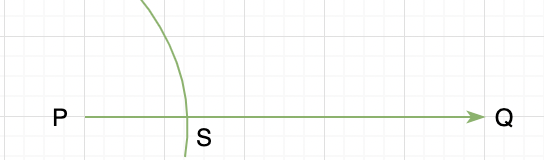Step 2. Now take S as the centre and the same radius as above. Draw an arc on the arc already drawn. Let’s say the point of intersection of both arcs is T.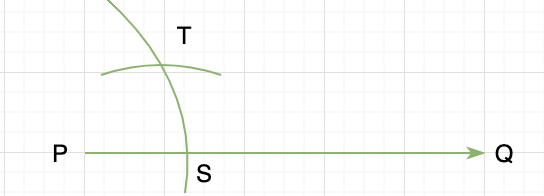Step 3. Draw a ray PR passing through T. This will give us the required angle of 60°.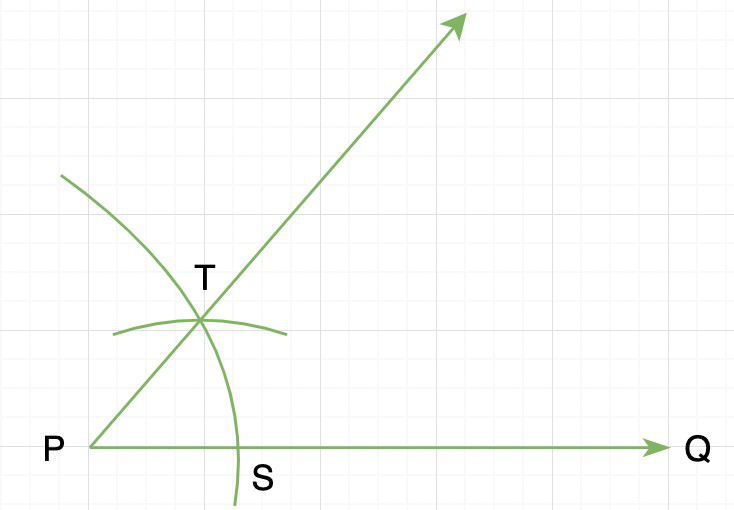Let’s see some examples on these concepts.

### Sample Problems

Question 1: In the figure below, ∠PQR is divided into many parts.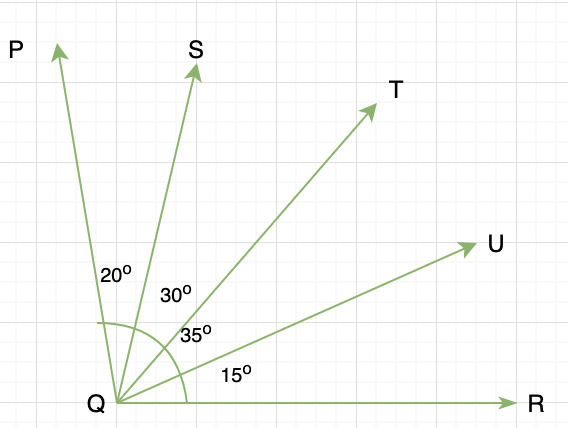Determine the bisector for ∠PQR.

Solution:

We know that bisector of an angle divides it into two equal parts.

Notice that,

∠PQT = ∠SQT + ∠PQS

∠PQT = 30° + 20°

∠PQT = 50°

and,

∠TQR = ∠TQU + ∠UQR

∠TQR = 35° + 15°

∠TQR = 50°

This the ray QT is the bisector of the angle PQR.

Question 2: State True or False for the statements below.

1. Perpendicular bisector bisects a line segment.
2. An angle bisector divides an angle into two equal parts.

Solution:

Statement 1: False

According to the definition of perpendicular bisector, it is a line that divides a line segment into two equal parts and is also perpendicular to it.

Statement 2: True

An angle bisector divides an angle into two equal parts.

Question 3: Construct an angle of 30° using the construction techniques mentioned above.

Solution:

Steps of Construction:

Step 1. Using the construction technique mentioned above for creating 60° angle. Let’s call that angle ∠CAB.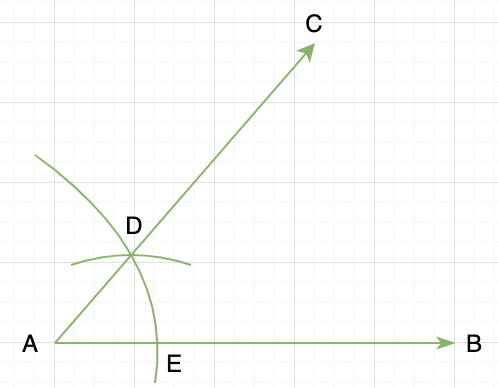Step 2. Now, since the angle is 60° and out goal is to construct an angle of 30°. We need to bisect this angle using the technique mentioned above.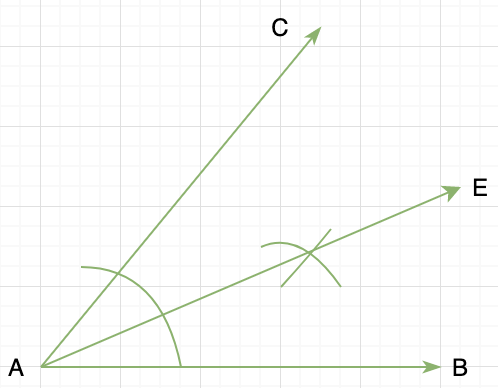Now the ray AE gives us the angle of 30°.

Question 4: Construct an angle of 135°.

Solution:

An angle of 135° can be made using one 90° and one 45°.

Let’s make a straight line PQ with a point R in between.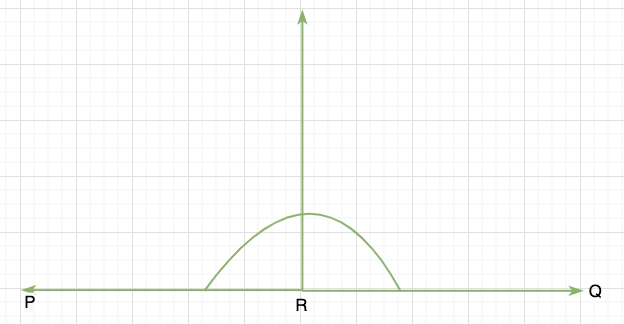Now we have made two 90° angles. We need to bisect on the of angles to make a 45°.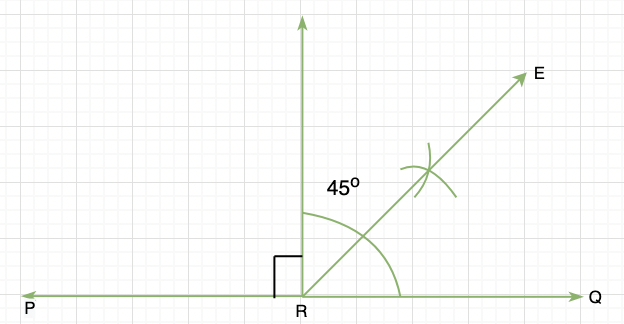Thus, ∠PRE gives us the required angle 135°.

My Personal Notes arrow_drop_up